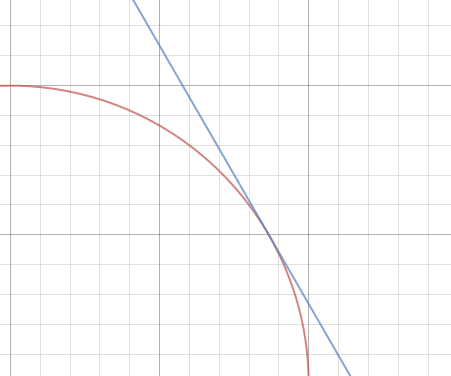# STOP CIRCLING AROUND

Geometry Level 2You have a circle which is based on the function $x^{2} + y^{2} = 1$. A line is tangent to that circle on the point, ($\frac{\sqrt{3}}{2},\frac{1}{2})$. What is the y-intercept of the line tangent to the circle ?

×